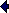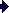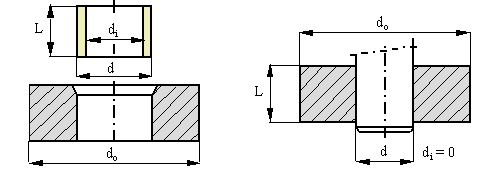Interference fitCalculate the interference pressure, friction force and friction torque.
di mm L mm  Ei GPa   vi
d mm δ/d  Eo GPa   vo
do mm µ
(Default value polymer bushing, steel hub)
interference pressure p MPa
friction force F kN
Transmission torque T Nm

 Stresses ring i ring o di d d do σt MPa MPa MPa MPa σr MPa MPa MPa MPa σe MPa MPa MPa MPa

Compute cooling temperature dTi respectively heating temperature dTo
interference δ/d for mounting
thermal expansion coefficient αi 10-6 K-1
thermal expansion coefficient αo 10-6 K-1

temperature decrease inner part for expansion fit dTi K
temperature increase outer part for shrink fit dTo K
 Symbols Ei modulus of elasticity body i [Pa] vi Poisson's ratio body i [ - ] dT1 temperature decrease inner part for expansion fit [K] dT2 temperature increase outer part for shrink fit [K] p interferencial pressure [Pa] F axial press fit force / tangential force to slip [N] T transmission torque [Nm] αi thermal expansion body i [m/mK] d diameter bore [m] δ diametral interference between both parts / d [ - ] L bearing length [m] µ coefficient of friction between both parts [ - ]